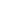Various forms of the Fourier series description for periodic signals are based on alternate ways of writing a cosine signal. Considerwith amplitude a > 0, frequency w > 0, and radian phase angle q . (The case of negative amplitude is treated by adding p  to q ). Three additional expressions for x(t) are (writing)

Trigonometric: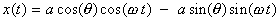Complex exponential: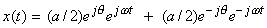Phasor real part: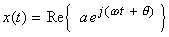Equivalence of these expressions can be verified by using  Euler's formula,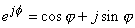and standard trigonometric identities.

We can visually explore properties of Fourier series using the phasor representation. A phasor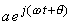can be viewed as a vector at the origin of the complex plane having length a  and, at any time  t, angle (w t + q ). The vector rotates counterclockwise with time, since w > 0, and the projection on the real axis is described by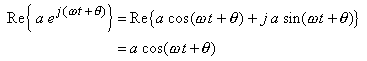For graphical representation, projection on the real (horizontal) axis is inconvenient, and therefore we rotate phasors by p/2  radians and project on the vertical axis. This makes use of the mathematical relationship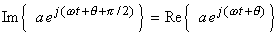Click on the complex plane below to define a vector of length  a  and initial angle, at t = 0, of  (q  + p/2) radians. Two periods of the corresponding phasor and cosine waveform will be shown with a convenient frequency w.

In a similar fashion, a sum of cosine waveforms can be represented as the real part of a phasor sum: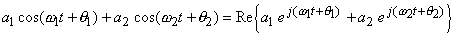The sum of two vectors in the complex plane can be found by placing the vectors “head to tail.” Click twice on the complex plane below to define a vector sum, and two periods of the corresponding two-cosine waveform with radian frequencies of w  and  2w  will be displayed.

Harmonic Phasor Sums

A sum of cosine signals with the property that every frequency is a nonnegative integer multiple of a fundamental frequencygenerates a periodic signal. This is because since each term in the sum repeats, at least once, in any time interval of length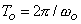. The terms in such a sum are said to be harmonically related. Simple examples arew(t) = 4 cos(3t) +  2 cos(6t + p/4)

which has fundamental frequency 3, and

y(t) = 2  + 4 cos(3t) +  2 cos(4t + p/4)

which has fundamental frequency 1. However,

z(t) = 1 + 4 cos(t) +  2 cos(p t)

is not a periodic signal since the frequencies 1 and p cannot be written as integer multiples of a single fundamental frequency.

Of course, any sum of harmonically related cosine signals can be viewed as the real part of a sum of harmonically related phasors. Using the applet below you can generate periodic signals by defining a sum of phasors. Each click on the complex plane defines the amplitude and phase angle for an additional harmonic phasor in the sum.

Fourier Series

Given a periodic signal x(t), straightforward calculations can be used to find the amplitude and phase angle coefficients in the Fourier series representation for x(t):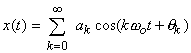If x(t) has  period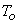, the fundamental frequency is given by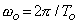, and we can assume that every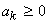. Terminology for the various terms is listed below:

·        dc-term, or zeroth harmonic term: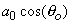·        fundamental frequency term:·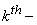harmonic, k ³ 1, term:The corresponding phasor representation for the Fourier series has the formOf course in practice the infinite sum is truncated to a finite number of terms. The applet below presents truncated Fourier series for a triangular wave, a square wave, and a periodic train of impulses. You can explore the effect of using various numbers of terms in the representation.The first few terms of these Fourier series are given by

·        triangular wave: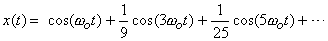·        square wave:·        impulse train:For a periodic signal that is a continuous function of time, such as the triangular wave, the Fourier series coefficients diminish at least as fast as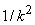. That is, for some positive constant M,You can observe in the applet above that it takes very few terms to achieve a good approximation to the triangular wave.

Gibbs Effect

For a function that is continuous except for a finite number of finite jumps in one period, such as the square wave, the coefficients diminish as 1/k. This raises mathematical issues of convergence, particularly at points of discontinuity, and gives rise to the Gibbs effect. The Gibbs effect is the overshoot phenomenon exhibited by the truncated Fourier series at points of discontinuity. This behavior is apparent with the square wave in the applet. Notice that as more terms are added to the Fourier series, the overshoot near a discontinuity decreases only slightly in amplitude, though it decreases significantly in duration.

A more extreme case is the impulse train, where the signal is a generalized function, and the Fourier series coefficients remain constant. The mathematical nature of convergence of the series is far from apparent. However, from the truncated series in the applet, it is clear that the Gibbs effect is present, and also that some type of convergence might be possible.

There are several methods for modifying the coefficients in a truncated Fourier series to reduce or eliminate Gibbs effect. Essentially these are different ways of weighting (decreasing) the coefficient values, and are referred to as windowing methods. One simple method is the Fejer Window, based on Fejer summation of series. In this method, if N harmonics are included in the truncated Fourier series, then the amplitude of the kth harmonic is multiplied by (N - k)/N. Thus including the first 5 harmonics (some have zero amplitude) for the square wave and impulse train yields the expressions

·        Fejer Window (N = 5) square wave: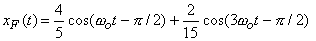·        Fejer Window (N = 5) impulse train:A second method is the Hamming Window, where the kth harmonic in an N harmonic series is multiplied by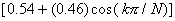For the square wave and impulse train examples, this yields the expressions

·        Hamming Window (N = 5) square wave: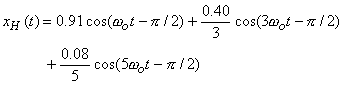·        Hamming Window (N = 5) impulse train:Notice that both windows apply a unity multiplier to the dc-term (k = 0), and that as N increases the multiplier on the kth-term tends to unity. Therefore the limit function of the series is unchanged. The success of these two approaches in eliminating the Gibbs effect can be explored in the applet below.  Also, there are a number of other windows that have been devised by choosing the weights according to various criteria.return to demonstrations page

 Applets by Hsi Chen Lee